Order Form WR
Multiple patients, or group orders allowed.
Each patient must submit certification.
1 pound maximum per order
•• - -
• Must submit valid identification.
•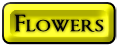•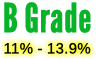\$2.01 to \$4.71 per gram
•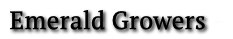• Not for the seasoned user.
•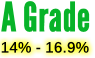•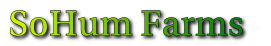• \$2.69 to \$4.71 per gram
•• \$2.45 to \$5.42 per gram
•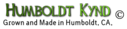• \$2.23 to \$5.07 per gram
•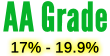\$3.33 to \$6.85 per gram
••\$3.18 to \$5.78 per gram
•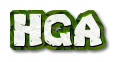• \$2.31 to \$5.42 per gram
•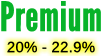•• \$3.66 to \$7.57 per gram
• \$4.96 to \$7.57 per gram
• \$2.87 to \$6.85 per gram
• \$3.66 to \$7.57 per gram
• \$3.66 to \$7.57 per gram
• \$3.11 to \$7.57 per gram
•\$3.44 to \$7.57 per gram
•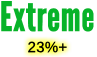•• \$4.55 to \$8.28 per gram
• \$5.21 to \$8.28 per gram
•• \$3.99 to \$8.28 per gram
• \$3.99 to \$8.28 per gram
• \$4.55 to \$8.28 per gram
• \$3.99 to \$8.28 per gram
••\$3.99 to \$8.28 per gram
•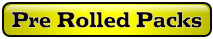•• \$5.67 to \$7.07 each
• \$4.25 to \$5.64 each
• \$6.75 to \$8.14 each
• \$5.67 to \$7.07 each
• \$8.22 to \$10.80 per gram
• \$8.22 to \$10.80 per gram
•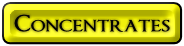•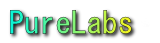• \$4.03 to \$8.28 per gram
• \$6.56 to \$11.14 per gram
• \$6.56 to \$11.14 per gram
• \$7.93 to \$11.85 per gram
•• \$6.56 to \$11.14 per gram
• \$6.56 to \$11.14 per gram
•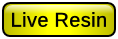One Gram Jars
•\$16.80 to \$26.00 per gram
• \$35.40 to \$40.00 per gram
• \$35.40 to \$40.00 per gram
• \$35.40 to \$40.00 per gram
• \$35.40 to \$40.00 per gram
•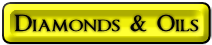One Gram Jars
•• \$34.75 to \$41.00 per gram
•• \$40.44 to \$56.00 per gram
•• \$21.14 to \$36.66 per gram
• \$7.48 to \$12.14 per gram
•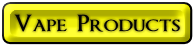C Cell Cartridges
•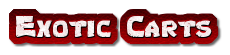\$12.30 to \$17.66 per gram
• \$11.90 to \$13.08 per gram
• \$6.04 to \$8.60 per gram
•\$21.50 to \$27.66 per gram
• Vape pen with charger
•\$21.80 to \$31.00 per gram
•C Cell Cartridges
•\$19.30 to \$25.00 per gram
•\$21.80 to \$31.00 per gram
•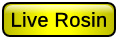C Cell Cartridges
•\$21.80 to \$31.00 per gram
•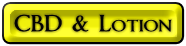•• \$2.22 to \$3.03 per gram
• \$2.22 to \$3.03 per gram
• \$2.22 to \$3.03 per gram
•• \$0.57 cents to 0.85 cents per gram
•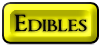•• \$3.28 to \$4.76 each
• \$4.03 to \$5.50 each
• \$4.03 to \$5.50 each
• \$20.31 to \$27.00 each
• \$0.79 cents to \$1.25 per gram
• \$1.15 cents to \$1.60 per gram
•• \$10.55 to \$12.80 each
• \$8.75 to \$17.50 each
• \$8.75 to \$17.50 each
• 8.75 to \$22.50 each
• \$12.75 to \$27.50 each
• \$12.75 to \$27.50 each
• \$12.80 to \$28.00 each
• \$15.75 to \$28.50 each
• \$15.75 to \$28.50 each
• \$15.75 to \$28.50 each
• \$15.75 to \$28.50 each
• \$15.75 to \$28.50 each
• \$15.75 to \$28.50 each
• \$15.75 to \$28.50 each
• \$15.80 to \$29.00 each
• \$15.80 to \$29.00 each
• \$4.33 to \$5.17 per gram
• \$4.33 to \$5.17 per gram
• \$2.70 to \$4.00 each
•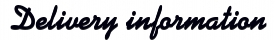Depending upon your location, delivery time varies from 2, up to 5 days, in extreme cases, or delays from extreme weather conditions.
• *USPS money order may delay delivery up to 2 days.
They can be difficult to cash.
• Total weight